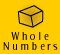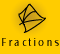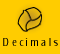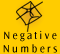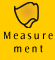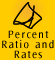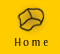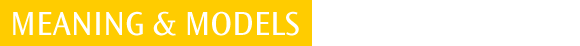| Percent - a special type of fraction | Percent Models | Ratios |
| Relationships:decimal fractions, common fractions, percent and ratio | Rates | Quick quiz |

Percent - a special type of fraction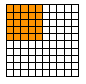0.25, 1/4, 25% These expressions tell us what portion of the square is coloured orange.

The word percent come from the expression 'per cent' and literally means 'a part of one hundred'. A percent is a part, or fraction, out of 100. For example:

 100% =100/100 =1 = 1.0 (decimal) 50% = 50/100 = 5/10 = 1/2 = 0.5 = 0.50 (decimal) 25% = 25/100 = 5/20 = 1/4 = 0.25 (decimal) 40% = 40/100 = 4/10 = 2/5 = 0.4 (decimal) 5% = 5/100 = 1/20 = 0.05 (decimal) 0.5% = 5/1000 = 1/200 = 0.005 (decimal)

We can see that to write a percent as a fraction we express the percent as a fraction with a denominator of 100. We may then be able to simplify the fraction further.

For example, 75% = 75/100 = 3/4

To express a fraction as a percent we must first convert the fraction into hundredths (in simple cases we can do this by using equivalent fractions) and then replace '/100' by the percent '%' sign.

For example, 4/5 = 80/100 = 80%

We can see that we express a percent as a decimal by dividing by 100.

For example,

 25% = 25/100 = 0.25 (twenty-five hundredths) 47.3 % = 47.3/100 = 0.473 (forty seven hundredths and 3 thousandths) 200% = 200/100 = 2

To express a decimal as a percent we multiply the decimal number by 100.

For example,

 0.108 = 0.108 x 100 = 10.8% 0.75 = .75 x 100 = 75% 1.2 = 1.2 x 100 = 120%

Some percents expressed as fractions and decimals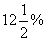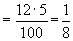= 0.125 (decimal)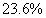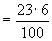= 0.236 (decimal)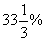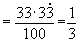= 0.333 (decimal, rounded to 3 decimal places)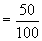= 0.5 = 0.50 (decimal)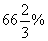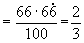= 0.667 (decimal, rounded to 3 decimal places)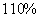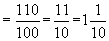= 1.1 (decimal)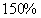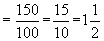= 1.5 (decimal)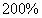=2.0 (decimal)

Example 1: 30 out of 50 apples in a box are too bruised to sell. What percent of apples cannot be sold?

 Working Out Thinking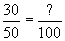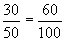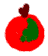30 out of 50 apples are bruised. To represent 30/50 as a percent we need to find out how many apples out of 100 are bruised. By equivalent fractions we know that 30 out of 50 equals 60 out of 100, so 60% of the apples are bruised. We could also say that, 3/5 of the apples are bruised 0.6 of the apples are bruised.

Example 2: Ryan spent 25 minutes in the bank, 11 minutes of which was spent waiting in a queue. What percent of time did he spend waiting in the queue?

 Working Out Thinking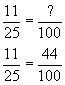Ryan spent 11 minutes out of 25 minutes waiting in a queue. To turn this into a percent we are asking, 11 out of 25 minutes equals how many minutes out of 100 minutes? We can see that 11 mins out of 25 mins equals 44 mins out of 100 mins by equivalent fractions (because we know 25 x 4 = 100) . We can say that Ryan spent 44%, 0.44 or 11/25 of his time in the bank waiting in a queue.

Example 3: What percent is 7 cm of 20 cm?

 Working Out Thinking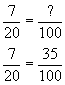To find out what percent 7 out of 20 is, we need to ask: 7 out of 20 is how many out of 100? 5 groups of 20 make 100, so 7 out of 20 is 35 out of 100 (5 x 7 out of 5 x 20). Therefore 7/20 equals 35%, or 0.35 if we represent it as a decimal.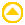Percent models

Dual-scale number line model

We can use the dual-scale number line, also called the proportional number line, to illustrate example 1 from above.

 Recall example 1: 30 out of 50 apples in a box are too bruised to sell. What percent of apples cannot be sold? Thinking The left side of the number line below has a percent scale. The right side of the number line has a number scale. We can label each scale using the information we are given in the problem.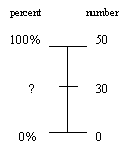We know that there are 50 apples in total, ie. 50 apples equals 100% of the apples. We know that 30 out of the 50 apples are bruised and we need to find what percent this is. In more complicated problems this dual-scale number line is a good way of organising the information we are given and to work out what information we need to find. Once we have represented the problem in this way we can write a proportion equation directly from the number line. 30/50 = ?/100 By equivalent fractions we know that 30/50 = 60/100. (Or we might have just noticed that it is a 'multiply by 2' relationship, so 30 x 2 = 60) Therefore 60% of apples are too bruised to sell.

The dual-scale number line model is discussed further in the other pages of the Percent, Ratio and Rates topic.

Elastic tape measure model

The tape measure model is a good linear model of percent. Teachers can easily make these models using a ruler, such as a 1 metre ruler, and elastic. The elastic needs to be marked with a percent scale. It can then be stretched to the desired length.

For example, what is 60% of 50?

To find the answer we line up the zeros of the ruler and the elastic. We then stretch the elastic so that 100% lines up with the whole amount, which in this case is 50. We then look for 60% on the elastic and read the corresponding amount on the ruler. We can see below that 60% of 50 is 30.

The intention is NOT to use this model accurately. It is a good way of showing that percent always involves a proportional comparison of something to 100.

 1 metre ruler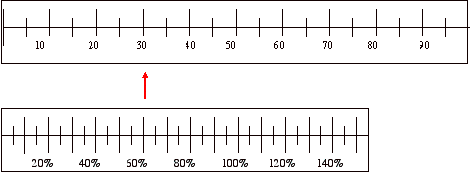Elastic

By manipulating the tape measure, this model can be used for the 3 types of percent problems, discussed in Percent Examples. Examples of which are,

What is 20% of 50?
What percent is 10 of 50?
30% of what number is 15?

(Note: for a lesson, a teacher will need elastics tape measures of various lengths, because the elastic can only be stretched - it cannot be shrunk).

(This elastic tape measure model was developed by J. H. Weibe)RatiosThe ratio of 1 : 3 tells us the ratio of shaded : unshaded The ratio of 3 : 1 tells us the ratio of unshaded : shaded The ratio of 1 : 4 tells us the ratio of shaded : whole

A ratio is another way of comparing quantities. Each quantity must be measured in the same units. An advantage of ratios is that we can compare several things at once.

 scale on a map 1:10000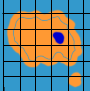(every 1 cm on the map represents 10000 cm on the ground, every inch on the map represents 10000 inches on the ground) ratio of blue to white paint is 1 : 4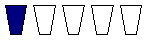(for every 1 litre of blue paint there are 4 litres of white paint; for every cup of blue paint there are 4 cups of white paint, i.e. 4 times as much white) ratio of gears on a bicycle 8 : 16 : 24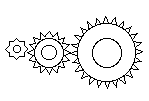8:16:24 teeth on each cog (cog size increases in the proportion of 1:2:3:4 etc) ratio of number of girls to number of boys in class is 5 : 2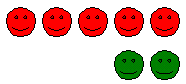(for every 5 girls in class there are 2 boys)

Although ratios must have each quantity measured in the same units, the units are not fixed. 1 litre of blue paint to 4 litres of the white paint represents the same ratio as 1 tin of blue paint to 4 tins of white paint, or 1 bucket of blue paint to 4 buckets of white paint. This fact makes ratios very versatile to use in everyday situations.

The order in which a ratio is written is very important. If we say the ratio of the number of girls to the number of boys is 5:2 this is very different to saying the ratio of the number of girls to the number of boys is 2:5.

A ratio can be written in different ways;

- in words - the ratio of the number of girls to the number of boys is 5 to 2, and this is the way we say it

- using a colon - number of girls : number of boys = 5 : 2

 We must always talk about ratios in context. To say or write 5 : 2, for example, has no meaning on its own.

Example 4: Let's say I want to make the paint colour 'sky blue' and I know that the way to do this is to mix 1 part blue with 4 parts white. This means there is a ratio of blue to white of 1:4. In this case 1 litre of blue to 4 litres of white, making 5 litres of sky blue paint.1 blue : 4 white 2 blue : 8 white

To make double the quantity of paint I can mix the blue to white as a ratio of 2:8. This will make the same colour. The ratios of 1:4 and 2:8 are equivalent, and worked out in the same way as equivalent fractions. We multiply each part of the original ratio by the same number and we can find equivalent ratios.

 1 : 4 1 : 4 1 : 4 x 2 x 3 x4 2 : 8 3 : 12 4 : 20

Sharing quantities in a given ratio

Example 5: We have a small inheritance of \$15000 to be shared among 3 people in the ratio of 2 : 2 : 1, how much does each person receive?

Working Out Thinking
 Person 1 Person 2 Person 3 2 : 2 : 1 (2 x \$3000) (2 x \$3000) (1 x \$3000) \$6000 \$6000 \$3000

The ratio of 2 : 2 : 1 means that the inheritance is divided into 5 portions - two people each receive 2 portions and one person receives 1 portion.

\$15000 divided by 5 - each portion is worth \$3000Relationships - decimal fractions, common fractions, percent and ratio

We can use examples to illustrate the relationships between decimal fractions, common fractions, percent and ratio.

Example 6: An inheritance of \$15000 is to be distributed among 3 people in the ratio of 2 : 2 : 1. (\$15000 will be divided into 5 portions)

 Person 1 Person 2 Person 3 ratio 2 : (2 x \$3000) 2 : (2 x \$3000) 1 (1 x \$3000) common fraction(of \$15000)(of \$15000)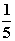(of \$15000) decimal fraction 0.4 (of \$15000) 0.4 (of \$15000) 0.2 (of \$15000) percent 40 % (of \$15000) 40 % (of \$15000) 20 % (of \$15000)

Example 7: Ratios and fractional parts.
A litre of mixed cordial requires 250 mls of cordial and 750 mls of water. How can we represent this as a ratio and a fraction?

 Thinking The ratio of cordial to water is 250 : 750 or 1 : 3. One part cordial to 3 parts water. In fraction terms, the 1000 mls of mixed cordial is 250/1000 (1/4) cordial and 750/1000 (3/4) water. We talk about the ratio of cordial to water in many different ways: For every cup of cordial there are 3 cups of water. There is 3 times as much water as cordial. 1 out of every 4 parts of the mixed cordial is cordial. Can you think of any more ways?

Example 8: Ratios and fractional parts.
A group of 100 people is made up of 60 males and 40 females. How can we represent this as a ratio and a fraction?

 Thinking The ratio of males to females is 60 : 40 or 6 : 4. This means that overall there is a higher proportion of males in the group, and for every 6 males there are 4 females. In fraction terms, where we are talking about the group of 100 people, 60/100 (6/10) are male and 40/100 (4/10) are female. So we can also say that 6 out of every 10 people are males and 4 out of every 10 people are females. Whereas fractions only enable us to represent the part to whole relationship (in this case, males/people and females/people), different aspects of the relationships between quantities (people) can be shown using ratios. For example, The 3 ratios that represent the relationships of males and females in this group of people are: - the ratio of 6 males to 10 people can be represented as 6 : 10 - the ratio of 4 females to 10 people can be represented as 4 : 10 - for every 6 males there are 4 females can be represented as 6 : 4 Which ratio we choose depends on we want to say. The number of males to the number of females is 6 : 4 The number of males to the number of people is 6 : 10 The number of females to the number of people is 4 : 10

In the early stages of introducing ratio at a primary level we generally discuss ratios in terms of a part to part or, quantity to quantity, comparison. At this stage part to whole relationships are often better represented by fractions or percents with which students already have some experience. When part to whole ratios are introduced care must be taken to ensure students clearly understand what is being represented.Rates

We use rates when we are measuring one quantity or amount in relation to another quantity or amount. We use them to compare how quantities change, usually over a period of time. A significant difference between rates and ratios is that when we are forming rates, each quantity is measured in different units to form new composite units.

For example, let's say you are travelling at a rate of 60 kilometres per hour (km/h). Here we are measuring kilometres in relation to hours and the rate unit becomes 'kilometres per hour', often written as km/h.

Other examples of rates are, an athlete running at 10 metres per second (m/s), and a factory using water at a rate of 450 litres per hour (l/h).

Some rates we commonly use are:

 km/h kilometres per hour c/L cents per litre \$/m dollars per minute c/m cents per minute

Example 9: A hose is running water at the constant rate of 100 litres an hour.
1. How many litres will run in 2 hours?
2. How many hours will it take to run 350 litres?

 Working Out Thinking 100 litres/1 hour = ? litres /2 hours 200 litres will run in 2 hours. We know that 100 litres runs in 1 hour and we need to find out how many litres runs in 2 hours. We can write this as litres/hour because this is what we are trying to find out. By equivalent fractions we know that 100/1 = 200/2. Therefore 200 litres will run in 2 hours.

 Working Out Thinking 100 litres/1 hour = 350 litres /? hours 350 litres/ ? hours = 100 litres /1 hour 350 litres/3.5 hours = 100 litres/1 hour We know that 100 litres runs in 1 hour and we need to know how long it takes to run 350 litres. 350 litres is 3.5 times 100 litres, so it takes 3.5 times as long i.e. 3.5 hours. Therefore 350 litres will run in 3.5 hours.Quick quiz

 1. Express the following percents as fractions and decimals: a) 95% b) 13.5% c) 42% d) 1% e) 0.1% 2. Express the following fractions as percents: a) 37/100 b) 164/100 c) 25/50 d) 14/20 e) 16/25 3. Express the following decimals as percents: a) 0.01 b) 0.83 c) 0.005 d) 1.10 e) 0.2 4. Express the following quantities as ratios: a) There were 3 boys for every 5 girls at school assembly. b) Nine people out of every ten watch television every night. c) In a class of 25 students 3 are left handed and 22 are right handed. d) To cook rice you need 1 cup of rice to 2 cups of water. e) To make ANZAC biscuits, you add the same amount of flour to sugar. 5. The following ratios of pets owned have been obtained from surveys of 5 local neighbourhoods. Find at least one equivalent ratio for each of the following: a) dogs : cats = 10 : 20 b) cats : dogs = 30 : 50 c) dogs : cats = 100 : 100 d) dogs : cats : guinea pigs = 6 : 4 : 2 e) fish : turtles = 75 : 25 6. Express each part-to-part ratio below as a common fraction, decimal fraction and a percent of the whole: a) men : women : children = 3 : 3 : 4 b) men : women : children = 11 : 4 : 5 c) adults : children = 4 : 1 d) men : women : children : pets = 5 : 3 : 6 : 1 7. Write each of these sentences as a rate: a) She ran 100 metres in 15 seconds. b) The bus travelled 850 kilometres in 10 hours. c) Ilana can type 160 words in 2 minutes. d) The factory packaged 600 packets of biscuits in 5 minutes.

Extra questions:

(a) What percentage of the following shape is shaded?

(b) What percentage is not shaded?

3. What is the ratio of pink to white in the following figure?4. A biro is 23 cm long and a ruler is 30 cm long.

(a) What is the ratio of the biro to the ruler?

(b) What is the ratio of the ruler to the biro?

5. Complete the following table.

 Percentage Common Fraction Decimal Fraction 32% 0.06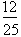6. A dog is three times as long as a bird. The dog is 90 cm long.

(a) How long is the bird?

(b) Write a ratio to show the relationship of the dog to the bird.

7. I have a 300g bag of sugar and a half a kilo of butter.

(a) What is the ratio of the sugar to the butter?

(b) What is the ratio of the butter to the sugar?

8. What two quantities are being measured when the units are km/hr?